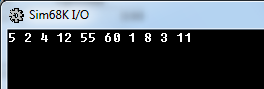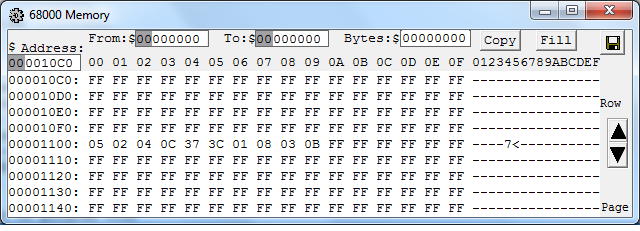Why indexing in an array starts at zero

By | July 15, 2014

Recently a curious intern at work asked why indexing in an array starts at zero. My explanation to him was that it pertains to offsets from the address of the start of the array. While this is an acceptable answer, it is not the only explanation as to why many programming languages (even those without pointer arithmetic) have adopted indexing of arrays starting at zero. I will begin by explaining my original answer and briefly go over a couple other compelling arguments.

Argument 1: “If Memory serves me right…”

The following will assume that the reader has some knowledge of what pointers are in the context of programming languages. Pointers are simply variables which store the address of another variable, hence they “point to” another variable. If that does not quite make sense to you then refer to either this C++ Pointer tutorial or the Wikipedia article on pointers.

I will begin this section with an example in C++. When we work with arrays in C/C++, the array name in most cases will decay into a pointer to the first element of the array. This does not mean that the array name itself is a pointer! It is not! A counter example to this common misunderstanding is the `sizeof` operator where the array name is instead treated as the array itself and not a pointer to the first element. Although the array name can usually can be treated as a pointer to the first element of the array.

Now when we access the elements of an array in C/C++ we use the dereferencing operator known as the offset operator ([]). As a simple example of what I am referring to:

What some programmers might not realize is that the offset operator adds the number between the brackets to the address that is being dereferenced which is the array name (treated as a pointer). So the following snippet is equivalent to the previous snippet:

So at this point, it should be clear that when we access index 0 (ie. `myArray`) of an array we are really just dereferencing the pointer to the first element of the array offset by 0 (ie. `*(myArray + 0)`). For those of us who are either very tired or mathematically challenged, that is just dereferencing `myArray` being treated as the pointer to the first element of the array and thus obtaining the value of that first element.

Personally, the fact that arrays in C/C++ are just a series of elements placed in a contiguous block of memory never really stuck with me all that much until I programmed in assembly language. What is that I hear? You want me to break out the wonderful EASy68K IDE? Well okay then!

Here is a quick little Motorola 68000 assembly language program that I wrote to further bring home the point of array traversal via offsets in memory by stressing the fact that arrays are just a collection of elements neatly placed side-by-side in the computers memory. Assembly language also does a great job of pushing the semantics onto the programmer.

Now when you execute this program you will receive the following output:A really cool aspect of the EASy68K IDE is the fact that you can view the raw memory of your 68K program. It works very well for this topic. You can read that C/C++ arrays are just a series of elements placed in contiguous memory locations until you are blue in the face, but sometimes visuals can aid in cementing our understanding. So here is the array beginning at address \$1100 as declared in the code where each subsequent element is placed next to the previous.I hope that it is now clear why indexing in an array starts at zero, at least for C/C++. You may be asking why it needs to be this way in languages like C#, Java or Python where we could possibly get around this fact. I think that it has more to do with consistency than anything else. Personally I started out programming in C++, later when I began learning to program in Java and C# it did not require any new knowledge to make use of their collection classes. Languages like Java and C# strive to be easy for C/C++ developers to pick up. Indeed, there are languages that begin their indexing at 1, but the vast majority of them start at 0.

Argument 2: Hardware influencing the Software

Source from StackOverflow

The first of the two other arguments is connected to the design of digital systems.

The argument begins with the realization that for any base b, the first b^N positive integers are represented by exactly N digits (including leading zeros) only if numbering starts at 0. So for base 2, we can represent 2^4 = 16 digits using 4 bits when numbering starts at 0. Which makes sense given that the sixteenth positive integer is 15 (%1111), if we start numbering at 0. However, if we do not start numbering at 0, then the sixteenth number becomes 16 (%10000) which is now 5 digits!

The larger implication of all this is that computer memory addresses have 2^N cells addressed by N bits. If we start counting at 1, then 2^N cells would need N+1 address lines! You then need an entirely new bit to access just a single address! You could leave this last address inaccessible, but why would you want to do that? So there you have it, the design of digital systems to start numbering at 0 has influenced the software which runs on them.

Argument 3: Mathematically Favorable

The extraordinary computer scientist Edsger Dijkstra outlined an argument built upon what is the most mathematically logical way to index a given sequence of elements in this EWD manuscript. The argument goes like this:

We can represent a sequence of natural numbers starting at a and ending at b in four possible different ways:

1. a <= i < b + 1
2. a – 1 < i <= b
3. a <= i <= b
4. a – 1 < i < b - 1

Let us start with the low hanging fruit. Number Three has the issue where writing an empty sequence is downright ugly. We can not simply write a <= i <= a as that is not empty (a is still in the sequence), so therefore we have to write a <= i <= a - 1 to achieve an empty sequence. Furthermore, if we assume that a is the smallest natural number, then our upper bound has now become an unnatural number! This option just went from ugly to hideous and therefore Number Three is out of the running.

Number Two and Number Four ultimately share the same fate. Say we choose to start our sequence at the smallest natural number, by the lack of the inclusion of the lower bound we force the lower bound into the realm of the unnatural numbers. For example, if a were the smallest natural number, then we would represent the lower bound as a – 1 < i ... which we can conclude that the number smaller than the smallest natural number is indeed an unnatural number.

Well that leaves Number One and although Harry Nilsson sings that it is the loneliest number, it is also the winner of this competition. Number One does not contain any of the undesirable traits of it’s brethren. It also has the added bonus that the difference between the bounds is the length of the sequence (Number Two has this trait as well).

From here we can index each element in the sequence of length N via a subscript (ordinal) in ideally one of two ways:

1. 1 <= i < N + 1
2. 0 <= i < N

Dijkstra argues (and I agree) that 0 <= i < N is the cleaner solution of these two for a couple of reasons. First, the ordinal of the element represents the number of elements preceding it in the sequence. Secondly, using N + 1 to represent the upper bound is a loss of elegance. You can easily inspect the 0 <= i < N representation to see that it is a sequence of length N.

So this concludes the discussion. Agree or disagree, these are just some of the common arguments for indexing an array starting at zero.